# Liquid Drop Model of Nucleus

Scattering experiments suggest that nuclei have approximately constant density, so that the nuclear radius can be calculated by using that density as if the nucleus were a drop of a uniform liquid. A liquid drop model of the nucleus would take into account the fact that the forces on the nucleons on the surface are different from those on nucleons on the interior where they are completely surrounded by other attracting nucleons. This is something similar to taking account of surface tension as a contributor to the energy of a tiny liquid drop. The volume of the liquid drop is proportional to the mass number A, and the surface would then be proportional to the two-thirds power of A.

The first step toward a liquid drop model of the nucleus would then be to postulate a volume term and a surface term in the form: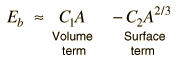This simple model in fact gives a reasonable approximation of the variation of nuclear binding energy with mass number when the constants have the values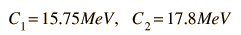Another contribution to the binding energy would be the coulomb repulsion of the protons, so there should be a negative term proportional to the square of the atomic number Z :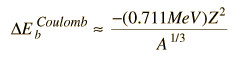The Pauli principle favors nuclei in which A=2Z, so the empirical model of binding energy contains a term of the form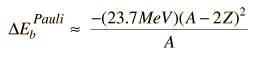Discussion of Pauli contribution

The Pauli principle also favors nuclear configurations with even numbers of neutrons and protons. In the liquid drop model, this is included by using the even-odd nucleus as a reference and adding a correction term which is positive for even-even nuclei and negative for odd-odd nuclei. This strategy for modeling the nuclear binding energy is attributed to Weizsaecker and called the Weizsaecker formula.

 Weizsaecker formula
Index

Nuclear Structure Concepts

Reference
Rohlf
Sec 11.3

 HyperPhysics***** Nuclear R Nave
Go Back

# The Weizsaecker Formula

The Weizsaecker formula is an empirically refined form of the liquid drop model for the binding energy of nuclei. It is also referred to as the "semi-empirical mass formula" and the "Bethe-Weizsaecker formula". Expressed in terms of the mass number A and the atomic number Z for an even-odd nucleus, the Weizsaecker formula is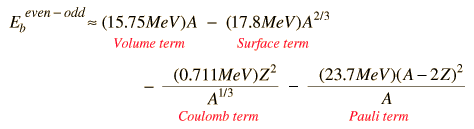Using the even-odd as a reference, there are then correction terms for even-even and odd-odd nuclei, the even-even groupings of protons and neutrons being favored in stability.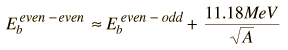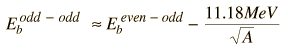An estimate of nuclear binding energy can then be obtained by first applying the even-odd formula.

 For atomic mass number A = , atomic number Z =
 and neutron number N = , the even-odd energy figure is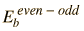= MeV

Then, if the nucleus is even-even or odd-odd, the appropriate correction can be made.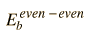= MeV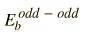= MeV

With the corrections, the estimate of the nuclear binding energy can be made.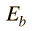= MeV

This binding energy can be checked by comparing it to the experimental nuclear binding energy in the nuclear data table accessible from the periodic table by clicking on the element and following the links.
 Liquid drop model
Index

Nuclear Structure Concepts

Reference
Rohlf
Sec 11.3

 HyperPhysics***** Nuclear R Nave
Go Back